Custom SearchPRACTICE PROBLEMS: Evaluate the following integrals: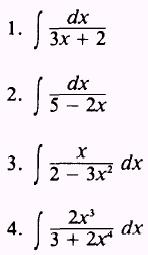ANSWERS: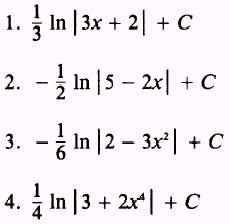METHOD 3 In the third method for solving integrals of quotients, we find that to integrate an algebraic function with a numerator that is not of lower degree than its denominator, we proceed as follows: Change the integrand into a polynomial plus a fraction by dividing the denominator into the numerator. After this is accomplished, apply the rules available. EXAMPLE: Evaluate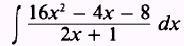SOLUTION: Divide the denominator into the numerator so that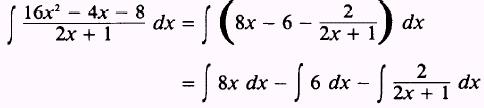Integrating each term separately, we have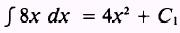and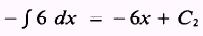andThen, by substitution, we find that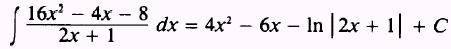where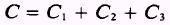EXAMPLE: EvaluateSOLUTION: The numerator in not of lower degree than the denominator; therefore, we divide and find that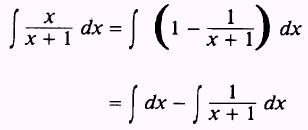Integrating each term separately, we find that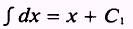and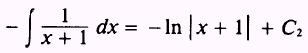Therefore,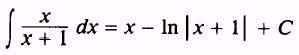wherePRACTICE PROBLEMS: Evaluate the following integrals: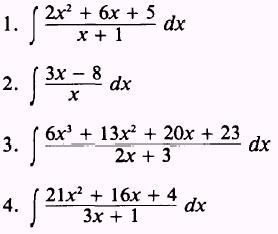ANSWERS:Integrated Publishing, Inc. - A (SDVOSB) Service Disabled Veteran Owned Small Business